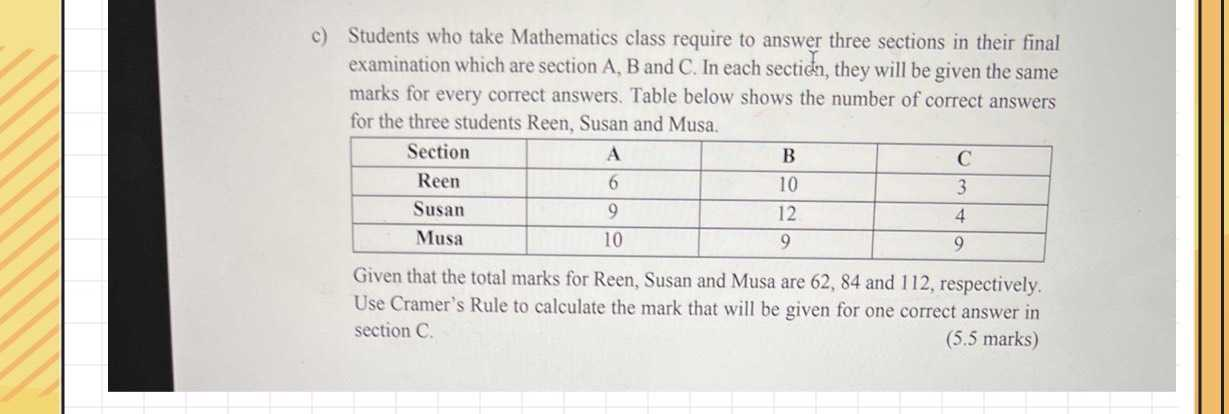### Still have math questions?

Algebra
QuestionStudents who take Mathematics class require to answer three sections in their final examination which are section $$A , B$$ and $$C$$ . In each section, they will be given the same marks for every correct answers. Table below shows the number of correct answers for the three students Reen, Susan and Musa.

Given that the total marks for Reen, Susan and Musa are $$62,84$$ and $$112$$ , respectively. Use Cramer's Rule to calculate the mark that will be given for one correct answer in section C.